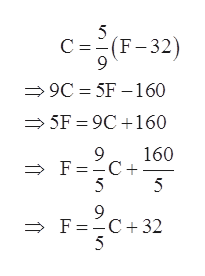# While on a tour in Greece, Tatyana saw that the temperature was 10 degrees Celsius. Solve for F in the formula C=5/9(F-32)F=

Question
21 views

While on a tour in Greece, Tatyana saw that the temperature was 10 degrees Celsius. Solve for F in the formula C=5/9(F-32)

F=

check_circle

Step 1

To convert 10 Celsius into Fahrenheit.

Step 2

Solving the given fo...help_outlineImage TranscriptioncloseC =-(F-32) 9. → 9C = 5F –160 = 5F = 9C +160 160 → F=-C+ 5 5 F=-C+32 > 5 fullscreen

### Want to see the full answer?

See Solution

#### Want to see this answer and more?

Solutions are written by subject experts who are available 24/7. Questions are typically answered within 1 hour.*

See Solution
*Response times may vary by subject and question.
Tagged in

### Equations and In-equations